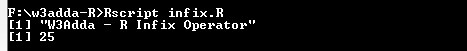# R Infix Function

In this tutorial you will learn about the R Infix Function and its application with practical example.

## R Infix Function

In R, generally functions are prefix, where function name comes before the argument list enclosed in parentheses, for example – fun(a,b). But with infix functions, the function name comes in between its arguments. For example, operators like + and – are actually infix functions, in fact these operators do a function call in the background. For instance, the expression

is actually converted its infix equivalent as

.Example:-

## Infix operators in R

Following is a list of predefined infix operators available in R –

 %% Remainder operator %/% Integer division %*% Matrix multiplication %o% Outer product %x% Kronecker product %in% Matching operator

## User defined infix operator

In R, a user-defined infix operator can be created as following –

Syntax:-

here, func_name is replaced with actual function name enclosed between percent sign(%). As percent sign(%) is a special character, you need to use it inside back ticks. Once an infix function is defined later it can invoked as following –

Syntax:-

Example:-

Output:-In this tutorial we have learn about the R Infix Function and its application with practical example. I hope you will like this tutorial.